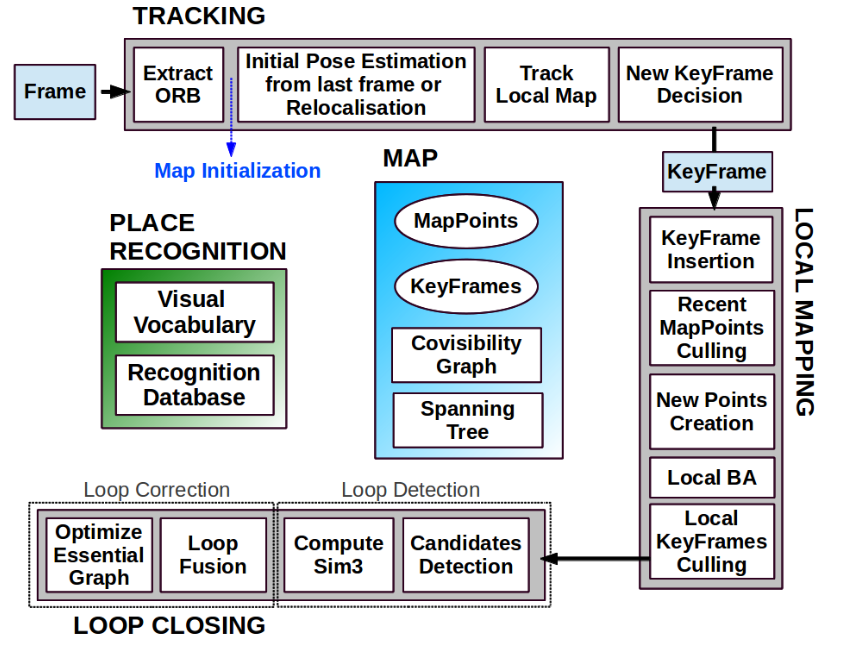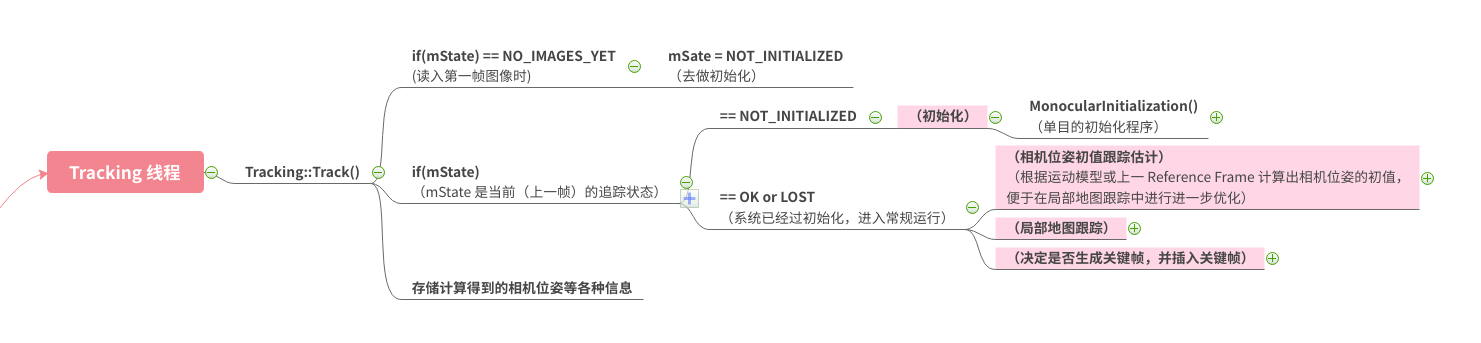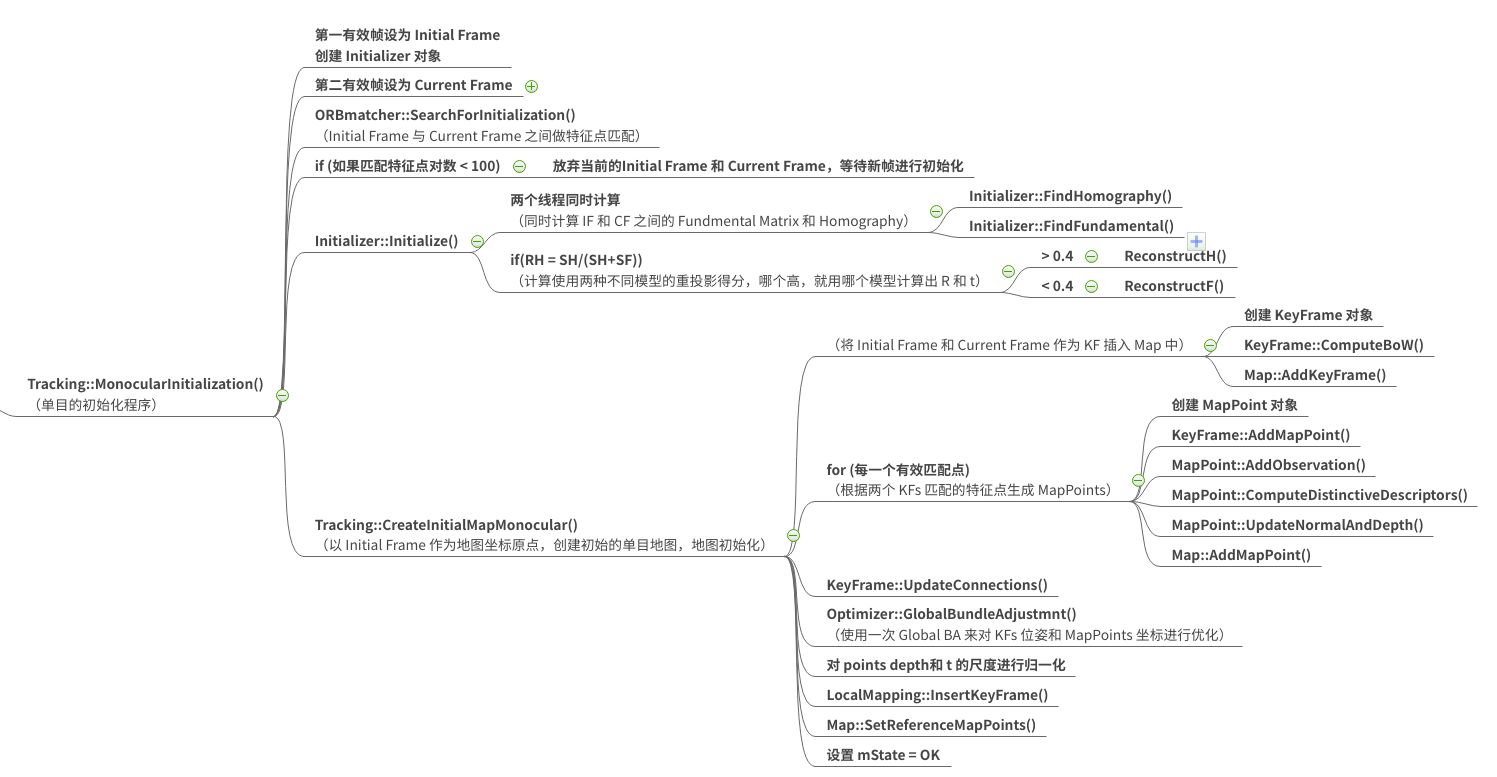# ORB-SLAM2 论文&代码学习 —— 单目初始化

• ORB-SLAM2 单目初始化部分 论文内容介绍
• ORB-SLAM2 单目初始化部分 代码结构介绍

# 写在前面# 以 ORB-SLAM 论文为参考

ORB-SLAM 在初始化时，它也不知道场景中的特征点在不在同一平面，所以它选择两种模型各自算一遍（开两个线程同时算），之后计算两种模型各自进行运动恢复的得分，取得分高的模型，再根据该模型，计算两帧之间的相对位姿并进行初始化。

## 单目初始化具体步骤

### 2. 同时计算两种模型

• 单应矩阵 $H_{cr}$：DLT 算法 + RANSAC 迭代
• 基础矩阵 $F_{cr}$：8点法 + RANSAC 迭代

$S_{M}=\sum_{i}\left(\rho_{M}\left(d_{c r}^{2}\left(\mathbf{x}_{c}^{i}, \mathbf{x}_{r}^{i}, M\right)\right)+\rho_{M}\left(d_{r c}^{2}\left(\mathbf{x}_{c}^{i}, \mathbf{x}_{r}^{i}, M\right)\right)\right)$

$\rho_{M}\left(d^{2}\right)=\left\{\begin{array}{ll} {\Gamma-d^{2}} & {\text { if } \quad d^{2}<T_{M}} \\ {0} & {\text { if } \quad d^{2} \geq T_{M}} \end{array}\right.$

### 3. 选择合适的模型

$R_{H}=\frac{S_{H}}{S_{H}+S_{F}}$

# 以 ORB-SLAM2 代码（程序导图为参考）PS： 从中上图我们可以看到，在恢复出两帧之间的相对运动后，程序中还要使用 Tracking::CreateInitialMapMonocular() 来建立初始化的地图。这部分在论文里几乎没有笔墨提到，但在程序里需要很大篇幅来实现。这个细节就反映了我在本系列博文开篇就提到过的读通 ORB-SLAM2 代码的困难之处。以我现在小菜鸡的水平，我根本想象不出 ORB-SLAM2 这样复杂而环环相扣的工程是怎么写出来的（流泪）。

# ORB-SLAM2 系列博文

ORB-SLAM2 系列博文

posted @ 2020-02-24 21:03  MingruiYu  阅读(722)  评论(0编辑  收藏